# Algebra II : Value of e

## Example Questions

### Example Question #1 : Factorials

What is the value of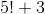.

Possible Answers: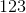None of the other answers.

Correct answer:Explanation:

When evaluating a factorial, you multiply the original number by each integer less than it, stopping at 1.

For this problem, this means that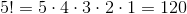.

Then adding 3, we get the answer 123

### Example Question #2 : Factorials

Which of the following best represents the approximate value of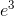?

Possible Answers:Correct answer:Explanation:

The value of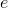is defined as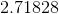.

To find, simply cube the decimal number.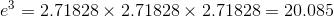The closest value of this number is:### All Algebra II Resources